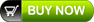# Topic Guides

FourQuants offers prepackaged topic guides consisting of downloadable PDF notes with EXCEL examples and templates.

The following is a list of topic guides are available:

 Asset Liability Management (ALM) Crash Course PDF notes ALM Crash Course Value at Risk with Liquidity Premium EXCEL Worksheets ALM Crash Course – Excel Examples [includes Cost of Close Liquidity Gaps, Cost of Close Interest Rate Risk, Earnings at Risk, Market Value of Equity] Duration Convexity Example  Value at Risk with Liquidity Premium – EXCEL ExampleBasel & ICAAP PDF notes ICAAP – Overview & Core Concepts ICAAP Sample Report Template & Executive Summary Basel III – Liquidity FrameworkBlack-Derman-Toy (BDT) Interest Rate Model PDF notes How to construct a Black Derman Toy Model in EXCEL How to utilize results of a Black Derman Toy Model EXCEL Worksheets Black-Derman-Toy Model Construction – EXCEL Example How to utilize results of a Black Derman Toy Model – EXCEL ExampleCalculating Value at Risk (VaR) PDF notes Calculating VaR – Includes case study Value at Risk with Liquidity Premium EXCEL Worksheets Calculating VaR – EXCEL Example Portfolio VaR – EXCEL Example Value at Risk with Liquidity Premium – EXCEL ExampleConstructing Volatility Surfaces in EXCEL PDF notes Constructing Volatility Surfaces in EXCEL EXCEL Worksheets Local Volatility Surface Model -NVIDIACredit Analysis & Credit Process PDF notes Credit Analysis – First Course Credit Analysis – Financial Institution Credit Process EXCEL Worksheets Credit Analysis – Financial Institution – EXCEL ExampleDerivative Products PDF notes Derivatives Terminology Crash Course Derivative ProductsForward Prices and Forward Rates – Calculation reference & detailed examples PDF notes Calculating Forward Price and Forward Rates in EXCEL EXCEL Worksheets Calculating Forward Prices, Rates, YTM & FRA ValuesHeath Jarrow Merton (HJM) Interest Rate Model EXCEL Worksheets Pricing IRS – Module I – Term Structures EXCEL Example Heath Jarrow Merton – HJM 3 – Factor Interest Rate Model Principal Component Analysis – PCA – US Treasury Yield RatesInterest Rate Simulation Crash Course PDF notes Interest Rate Simulation Crash Course EXCEL Worksheets Pricing IRS – Module I – Term Structures EXCEL Example Calibration of CIR Model Example Black-Derman-Toy Model Construction – EXCEL Example How to utilize results of a Black Derman Toy Model – EXCEL Example Heath Jarrow Merton – HJM 3 – Factor Interest Rate Model Principal Component Analysis – PCA – US Treasury Yield RatesMonte Carlo Simulation EXCEL Worksheets Monte Carlo Simulation – Commodity – Example Monte Carlo Simulation – Currency – Example Monte Carlo Simulation – Equity – ExampleMonte Carlo Simulation with Option Pricing PDF Note Derivative Pricing Monte Carlo Simulation – Model and Applications EXCEL Worksheets Valuing Options – Traditional Binomial Trees – Examples Valuing Options – Black Scholes – Examples Derivative Pricing – Binomial Trees – Example Derivatives Pricing using Monte Carlo Simlator Pricing Ladder Options using Monte Carlo SimlatorPricing Interest Rate Swaps and Interest Rate Options PDF notes Pricing IRS – Module I – Term Structures Pricing IRS – Module II – IRS and CCS Pricing Interest Rate Options – Module III EXCEL Worksheets Pricing IRS – Module I – Term Structures EXCEL Example Pricing IRS – Module II – IRS and CCS EXCEL Example Pricing Interest Rate Options – Module III EXCEL ExampleSetting Counterparty Limits PDF notes Setting Counterparty Limits Sample Counterparty Limit Proposal EXCEL Worksheets Setting Limits – EXCEL ExampleTreasury Crash Course PDF notes Treasury Crash Course Value at Risk with Liquidity Premium EXCEL Worksheets ALM Crash Course – Excel Examples [includes Cost of Close Liquidity Gaps, Cost of Close Interest Rate Risk, Earnings at Risk, Market Value of Equity] Duration Convexity Example  Calculating VaR – EXCEL Example Value at Risk with Liquidity Premium – EXCEL ExampleUnderstanding Delta Hedging and Greeks PDF notes Understanding Delta Hedging and Greeks EXCEL Worksheets Delta Hedging – Call Option Delta Hedging – Put Option Greeks## Random Testimonial

• ~ Student Testimonials

"Concise, to the point. Very informative and very practical.”

“I loved the course!! Very interesting. Good lectures.”

“Excellent, very informative and inspiring.”

“In total awe of the way he taught…”

“Well versed with the subject and able to transmit the subject properly.”

“Excellent. If the time could be extended this would be an even better learning experience.”

Excellent teaching skills. The instructor made every effort to explain most complicated finance concepts in simplest possible ways."